6333.0 - Characteristics of Employment, Australia, August 2016
ARCHIVED ISSUE Released at 11:30 AM (CANBERRA TIME) 02/05/2017
 Page tools: .mffeedback,#pjs { display :none; } Enable Javascript to Print PagesPrint All Explanatory Notes Glossary Populations and Data items list (Appendix) Population concordance (Appendix) Distribution of earnings (Appendix) Data Cubes (I-Note) Data Quality (Technical Note) (Technical Note) Quality Declaration - Summary TECHNICAL NOTE DATA QUALITY INTRODUCTION 1 Since the estimates in this publication are based on information obtained from occupants of a sample of dwellings, they are subject to sampling variability. That is, they may differ from those estimates that would have been produced if all dwellings had been included in the survey. One measure of the likely difference is given by the standard error (SE), which indicates the extent to which an estimate might have varied by chance because only a sample of dwellings was included. There are about two chances in three (67%) that a sample estimate will differ by less than one SE from the number that would have been obtained if all dwellings had been included, and about 19 chances in 20 (95%) that the difference will be less than two SEs. 2 Another measure of the likely difference is the relative standard error (RSE), which is obtained by expressing the SE as a percentage of the estimate. RSE% = (SE/estimate ) x 100 3 RSEs for Characteristics of Employment estimates have been calculated using the Jackknife method of variance estimation. This process involves the calculation of 30 'replicate' estimates based on 30 different sub-samples of the original sample. The variability of estimates obtained from these sub-samples is used to estimate the sample variability surrounding the main estimate. 4 The Excel spreadsheets in the Downloads tab contain all the tables produced for this release and the calculated RSEs for each of the estimates. The RSEs for estimates other than medians have been calculated using the Jackknife method, and RSEs for the medians have been calculated using the Woodruff method. 5 In the tables in this publication, only estimates (numbers, percentages, means and medians) with RSEs less than 25% are considered sufficiently reliable for most purposes. However, estimates with larger RSEs have been included. Estimates with an RSE in the range 25% to 50% should be used with caution while estimates with RSEs greater than 50% are considered too unreliable for general use. All cells in the Excel spreadsheets with RSEs greater than 25% contain a comment indicating the size of the RSE. These cells can be identified by a red indicator in the corner of the cell. The comment appears when the mouse pointer hovers over the cell. CALCULATION OF STANDARD ERROR 6 RSEs are routinely presented as the measure of sampling error in this publication and related products. SEs can be calculated using the estimates (counts or means) and the corresponding RSEs. 7 An example of the calculation of the SE from an RSE follows. Datacube 4 shows that the estimated number of males aged 55–59 years who were employed part-time was 81,000 in August 2016, and the RSE for this estimate was 7.5%. The SE is: SE of estimate = (RSE / 100) x estimate = 0.075 x 81,000 = 6,100 (rounded to the nearest 100) 8 Therefore, there are about two chances in three that the value that would have been produced if all dwellings had been included in the survey would fall within the range 74,900 to 87,100 and about 19 chances in 20 that the value would fall within the range 68,800 to 93,200. This example is illustrated in the following diagram.PROPORTIONS AND PERCENTAGES 9 Proportions and percentages formed from the ratio of two estimates are also subject to sampling errors. The size of the error depends on the accuracy of both the numerator and the denominator. A formula to approximate the RSEs of proportions not provided in the spreadsheets is given below. This formula is only valid when x is a subset of y. 10 Considering Datacube 4, of the 1,532,300 males aged 25-34 years who were employed, 1,334,500 or 87.1% were full-time workers. The RSE of 1,334,500 is 1.2% and the RSE for 1,532,300 is 1.0%. Applying the above formula, the RSE for the proportion of males aged 25–34 years who were full-time workers: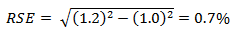11 Therefore, the SE for the proportion of males aged 25–34 years who were full-time workers was 0.6 percentage points (= (87.1/100) x 0.7). Therefore, there are about two chances in three that the proportion of males aged 25–34 years who were full-time workers is between 86.5% and 87.7%, and 19 chances in 20 that the proportion was within the range 85.9% to 88.3%. SUMS OR DIFFERENCES BETWEEN ESTIMATES 12 Published estimates may also be used to calculate the sum of two or more estimates, or the difference between two survey estimates (of numbers, means or percentages) where these are not provided in the spreadsheets. Such estimates are also subject to sampling error. 13 The sampling error of the difference between two estimates depends on their SEs and the relationship (correlation) between them. An approximate SE of the difference between two estimates (x–y) may be calculated by the following formula: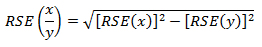14 The sampling error of the sum of two estimates is calculated in a similar way. An approximate SE of the sum of two estimates (x+y) may be calculated by the following formula: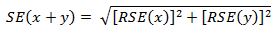15 Considering the example in paragraph 7, the estimated number of males aged 55–59 years who were employed part-time was 81,000, and the SE for this estimate was 6,100. From Datacube 4, the estimate of males aged 60-64 years who were employed part-time was 96,700 and the SE was 5,500. The estimate of males aged 55–64 years who were employed part-time is: 81,000 + 96,700 = 177,700 16 The SE of the estimate of males aged 55-64 years who were employed part-time is: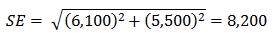17 Therefore, there are about two chances in three that the value that would have been produced if all dwellings had been included in the survey would fall within the range 169,500 to 185,900 and about 19 chances in 20 that the value would fall within the range 161,300 to 194,100. 18 While these formulae will only be exact for sums of, or differences between, separate and uncorrelated characteristics or subpopulations, it is expected to provide a good approximation for all sums or differences likely to be of interest in this publication. STANDARD ERRORS OF MEANS AND SUMS 19 The estimates of means and sums of continuous variables are subject to sampling variability and random adjustment. As for population estimates, the variability due to sampling and random adjustment is combined into the calculated Standard Error, and the Relative Standard Error is reported. The component of variability arising from sampling is calculated using the Jackknife method. STANDARD ERRORS OF QUANTILES 20 The estimates of quantiles such as medians, quartiles, quintiles and deciles are subject to sampling variability and random adjustment. As for population estimates, the variability due to sampling and random adjustment is combined into the calculated Standard Error, and the Relative Standard Error is reported. The component of variability arising from sampling is calculated using the Woodruff method. This is also true for Equal Distribution Quantiles. SIGNIFICANCE TESTING 21 A statistical test for any comparisons between estimates can be performed to determine whether it is likely that there is a significant difference between two corresponding population characteristics. The standard error of the difference between two corresponding estimates (x and y) can be calculated using the formula in paragraph 9. This standard error is then used to calculate the following test statistic: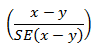22 If the value of this test statistic is greater than 1.96 then there is evidence, with a 95% level of confidence, of a statistically significant difference in the two populations with respect to that characteristic. Otherwise, it cannot be stated with confidence that there is a difference between the populations with respect to that characteristic. 23 The imprecision due to sampling variability, which is measured by the SE, should not be confused with inaccuracies that may occur because of imperfections in reporting by respondents and recording by interviewers, and errors made in coding and processing data. Inaccuracies of this kind are referred to as non-sampling error, and they occur in any enumeration, whether it be a full count or sample. Every effort is made to reduce non-sampling error to a minimum by careful design of questionnaires, intensive training and supervision of interviewers, and efficient operating procedures. Document Selection These documents will be presented in a new window.